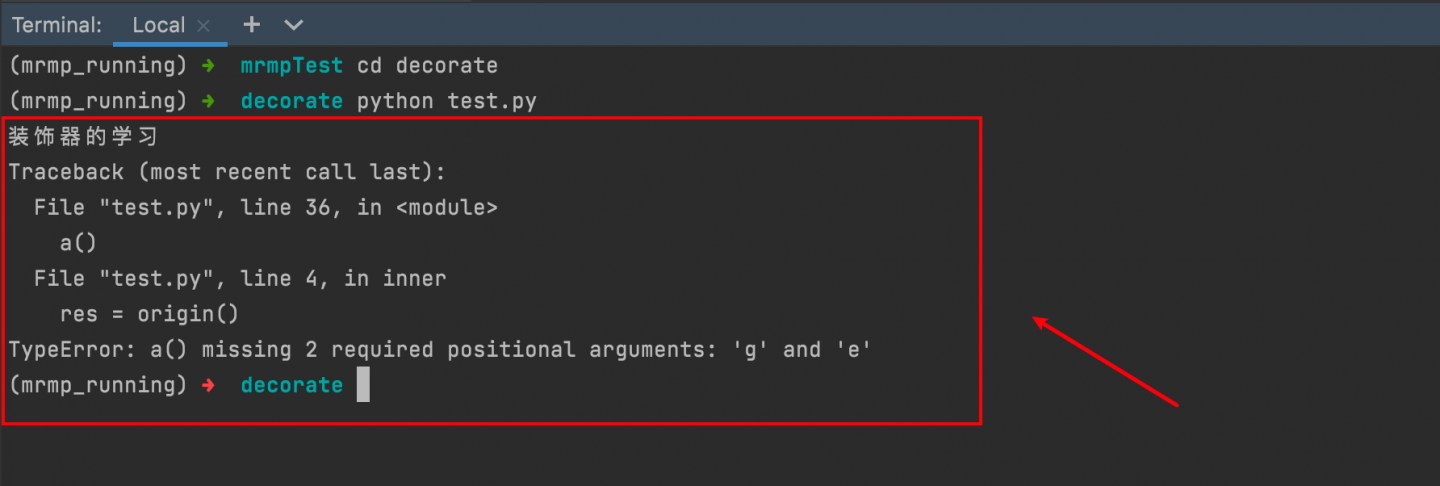# python 装饰器这一篇就够了

## 二、装饰器

### 装饰器的写法：

def a():
pass

def b():
pass

def c():
pass

def d():
pass

def e():
pass

def a():
print("装饰器的学习")
print("装饰器的学习")

def b():
print("装饰器的学习")
print("装饰器的学习")

def c():
print("装饰器的学习")
print("装饰器的学习")

def d():
print("装饰器的学习")
print("装饰器的学习")

def e():
print("装饰器的学习")
pass
print("装饰器的学习")

a()
b()
c()
d()
e()def outer(origin):
def inner():
print("装饰器的学习")
res = origin()
print("装饰器的学习")
return res

return inner

@outer
def a():
pass

@outer
def b():
pass

@outer
def c():
pass

@outer
def d():
pass

@outer
def e():
pass

a()
b()
c()
d()
e()• 首先：
我们要明白@的作用，那我们的函数a来举例子@的作用就是帮我们执行一次a=outer(a)，首先python将把我们的a变成参数传给outer函数，运行后再赋值给a，这就是@的作用。
• 其次给大家解释一下自定的outer函数
我自己称这个函数为@下函数的补丁函数，也就是装饰器函数还是拿a函数举例子，首先a函数变成参数传给了我们的outer函数，outer里又嵌套了一个inner函数 ，然后将函数a赋值给res，然后用return语句返回出结果，外层函数返回inner函数，也就是将inner函数运行一次，这就是工作流程。• 装饰器的参数
这时我遇到一个问题如果函数内有参数而且每个函数的参数数量不同，我们应该怎末办，先看下面代码
 def outer(origin):
def inner():
print("装饰器的学习")
res = origin()
print("装饰器的学习")
return res

return inner

@outer
def a(g, e):
pass

@outer
def b(w):
pass

@outer
def c(u, y, t):
pass

@outer
def d(c):
pass

@outer
def e():
pass

a()
b()
c()
d()
e()def outer(origin):
def inner(*args, **kwargs):
print("装饰器的学习")
res = origin(*args, **kwargs)
print("装饰器的学习")
return res

return inner

@outer
def a(a1):
print("我是一函数")

@outer
def b(a1, a2):
print("我是二函数")

@outer
def c(a5, a6, a7):
print("我是三函数")

a(1)
b(2, 3)
c(4, 5, 6)

### 装饰器的拓展：（functools模块）

| name |desc  |
| -------- | -------- |
| .__name__    | 获取函数名字     |
| .__doc__   | 获取函数内的注释     | 

def outer(origin):
def inner(*args, **kwargs):
# 我是一个装饰器函数
print("装饰器的学习")
res = origin(*args, **kwargs)
print("装饰器的学习")
return res

return inner

@outer
def c(a5, a6, a7):
# 我是个函数
print("我是三函数")

c(4, 5, 6)
print(c.__name__)
print(c.__doc__)

(myenv) ➜  decorate python test.py

inner
None
(mrmp_running) ➜  decorate


import functools

def outer(origin):
@functools.wraps(origin)
def inner(*args, **kwargs):
# 我是一个装饰器函数
print("装饰器的学习")
res = origin(*args, **kwargs)
print("装饰器的学习")
return res

return inner

@outer
def c(a5, a6, a7):
# 我是个函数
print("我是三函数")

c(4, 5, 6)
print(c.__name__)
print(c.__doc__)

 (myenv) ➜  decorate python test.py

c
None
(myenv) ➜  decorate


### 装饰器模板：

import functools

def outer(origin):
@functools.wraps(origin)
def inner(*args, **kwargs):
# 这里书写需要装饰的功能
res = origin(*args, **kwargs)
return res

return inner